# Ncert Chemistry Class 12 Questions Solutions Chapter 2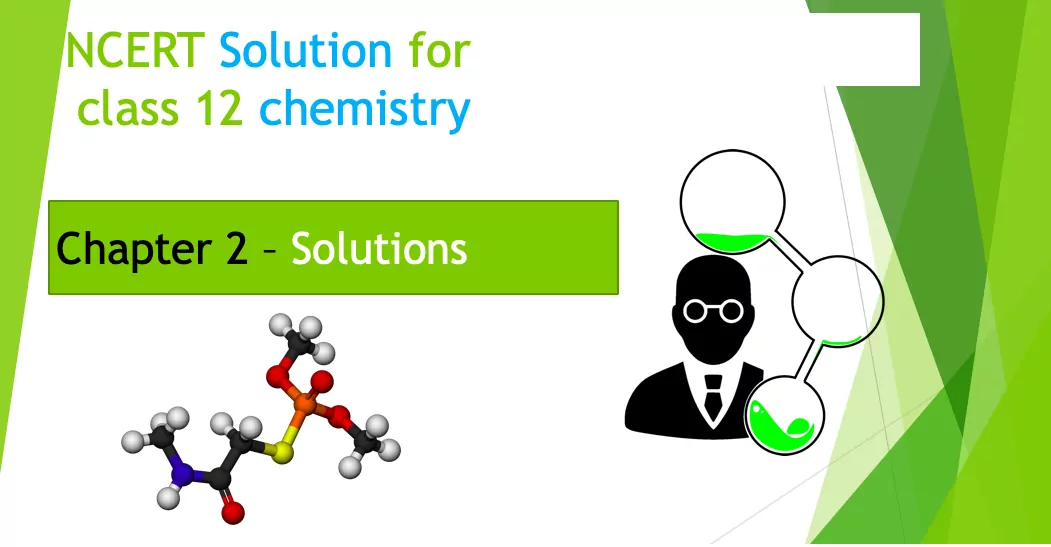Ncert Chemistry Class 12 Questions Solutions Chapter 2

NCERT
Intext Questions
Chapter – Solutions

Que 1. Calculate the mass percentage of benzene (C6H6) and carbon tetrachloride (CCI4) if 22 g of benzene is dissolved in 122 g of carbon tetrachloride.
Ans 1. Mass of solution = Mass of Benzene (C6H6) + Mass of Carbon Tetrachloride (CCl4)
= 22 g +122 g = 144 g
Mass percentage of Benzene = 22/144 × 100 =15.28 %
Mass % of CCl4 = 122/144 × 100 = 84.72 %

Que 2. Calculate the mole fraction of benzene in solution containing 30% by mass in carbon tetrachloride.
Ans 2. Let the mass of Solution = 100 g
∴ Mass of Benzene in the solution = 30 g
∴ Mass of Carbon Tetrachloride = 100 – 30g = 70g
Molar mass of Benzene (C6H6) = 78 g mol-1
Molar mass of CCl4 = 12 + 4 × 35.5 = 154 g mol-1
∴ No. of moles of benzene = 30/78 mol = = 0.3846 mol
Molar mass of CCl4 = 1 × 12 + 4 × 35.5 = 154 g/mol
Therefore, Number of moles of CCl4 = 70/154 = 0.4545 mol
Thus, the mole fraction of C6H6 is given as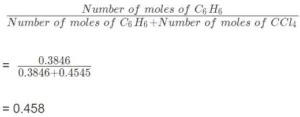Ncert Chemistry Class 12 Questions Solutions Chapter 2

Que 3. Calculate the molarity of each of the following solutions
(a) 30 g of Co(NO3)26H2O in 4·3 L of solution
(b) 30 mL of 0-5 M H2SO4 diluted to 500 mL.
Ans 3. We know that,
Molarity =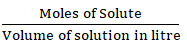(a) Molar mass of Co(NO)3.6H2O = 59 + 2 (14 + 3 × 16) + 6 × 18 = 291 g/mol
Therefore, Moles of Co(NO)3.6H2O = 30/291  mol
= 0.103 mol
Therefore, Molarity = 0.1o3/4.3 mol/lt = 0.023 M

(b) Number of moles present in 1000 mL of 0.5 M H2SO4 = 0.5 mol
Therefore, Number of moles present in 30 mL of 0.5 M H2SO4 =  0.5 × (30/1000)mol
= 0.015 mol
Therefore, Molarity = 0.015/0.5 L = 0.03 M

Que 4. Calculate the mass of urea (NH2CONH2) required in making 2.5 kg of 0.25 molal aqueous solution.
Ans 4. Moles of urea = 0.25 mole
Mass of solvent (water) = 1 kg = 1000 g
Molar mass of urea (NH2CONH2) = 2(1 × 14 + 2 × 1) + 1 × 12 + 1 × 16 = 60 g/mol
∴ Mass of urea in 1000 g of water = 0.25 mol × 60 g mol-1 = 15 g
Total mass of solution = 1000 + 15 g = 1015 g
2.5 kg means 2500 g
1015 g of solution contain urea = 15 g
1 g of solution contains urea = 15/1015
Therefore, 2500 g of solution contains Urea = 15 × 2500/1015
= 37g

Que 5. Calculate (a) molality (b) molarity and (c) mole fraction of Kl if the density of 20% (mass/mass) aqueous Kl is 1.202 g mL.
Ans 5. (a).  Calculation of molality of the solution
Weight of KI in 100 g of the solution = 20 g
Weight of water in the solution = 100 – 20 = 80 g
Molar mass of KI = 39 + 127 = 166 g mol-1
Therefore, the molality of the solution
= moles of KI/Mass of water in Kg  = (20/166)/ 0.08
= 1.506 m
= 1.51 m

(b) It is given that the density of the solution = 1.202 g mL-1
Mass / Density = Volume of 100 g solution
100g / 1.202 gml-1
= 83.19 mL
= 83.19 × 10-3 L
Therefore, molarity of the solution = (20/166) / 83.19 × 10-3 L
= 1.45 M
(c) Moles of KI
Moles of water = 80/18 = 4.44
Therefore, the mole fraction of KI = moles of KI/moles of KI + moles of water = 0.12/0.12 + 4.44
= 0.0263

Ncert Chemistry Class 12 Questions Solutions Chapter 2

Que 6. H2S a toxic gas with a rotten egg-like smell is used for qualitative analysis. If the solubility of H2S in the water at STP is 0.195 m, calculate Henry’s law constant.
Ans 6. The solubility of H2S in the water at STP = 0.195 m
Mass of H2O = 1000 g
Number of moles of Water =  = 55.56 mol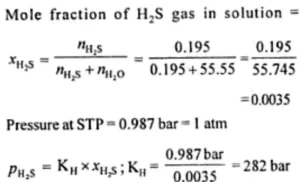Que 7. Henry’s law constant for CO2 in water is 1.67 × 108 Pa at 298 K. Calculate the quantity of CO2 in 500 mL of soda water when packed under 2.5 atm CO2 pressure at 298K.
Ans 7.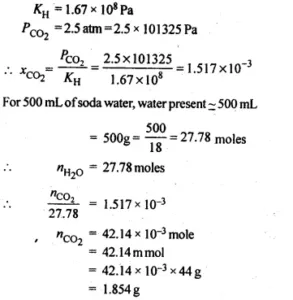Ncert Chemistry Class 12 Questions Solutions Chapter 2

Que 8. The vapour pressures of pure liquids A and B are 450 and 700 mm Hg at 350 K respectively. Find out the composition of the liquid mixture if the total vapour pressure is 600 mm Hg. Also, find the composition of the vapour phase.
Ans 8. P0A = 450 mm of Hg
P0B = 700 mm of Hg
Ptotal​ = 600 mm of Hg
According to Raoult’s law:
PA = P0AXA      PB = P0BXB
We know XA + XB = 1
= XB = 1 – XA
Therefor PB = P0B (1-XA)
Therefore, total pressure, PTotal = PA + PB
PTotal = P0AXA + P0B (1-XA)
PTotal = P0AXA + P0B – P0B XA
PTotal = (P0A – P0B)XA + P0B
600 = (450 – 700) XA + 700
250 XA = 100
XA = 100/250 = 0.4
Therefore, XB = 1 – XA = 1 – 0.4 = 0.6
PA = P0AXA = 450 × 0.4 = 180 mm of Hg
PB = P0BXB = 700 × 0.6 = 420 mm of Hg
Now, in the vapour phase: Mole fraction of liquid A = PA / PA + PB
= 180 / 180 + 420
= 180/600 = 0.30
And, mole fraction of liquid B = 1 – 0.30 = 0.70

Que 9. The Vapour pressure of pure water at 298 K is 23.8 mm Hg. 50 g of urea (NH2CONH2) is dissolved in 850 g of water. Calculate the vapour pressure of water for this solution and its relative lowering.
Ans 9.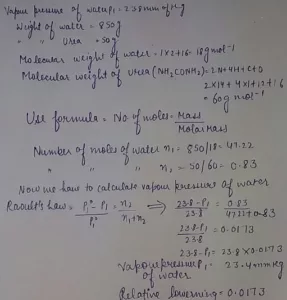Ncert Chemistry Class 12 Questions Solutions Chapter 2

Que 10. The boiling point of water at 750 mm Hg is 99.63°C. How much sucrose is to be added to 500 g of water such that it boils at 100°C? The Molal elevation constant for water is 0.52 K kg mol-1.
Ans 10.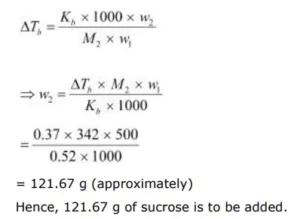Que 11. Calculate the mass of ascorbic acid (vitamin C, C6H8O6) to be dissolved in 75 g acetic acid to lower its melting point by 1.5°C, KF = 3.9 K kg mol-1.
Ans 11. Mass of acetic acid (w1) = 75 g
Molar mass of ascorbic acid (C6H8O6), M2­­ = 6 x 12 + 8 x 1 + 6 x 16 = 176 g mol-1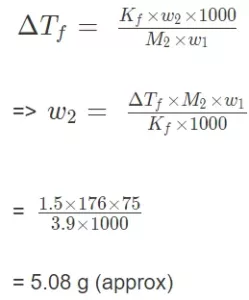Hence, the amount of ascorbic acid needed to be dissolved is 5.08 g.

Que 12. Calculate the osmotic pressure in pascals exerted by a solution prepared by dissolving 1.0 g of polymer of molar mass 185,000 in 450 mL of water at 37°C.
Ans 12. Volume of water (V) = 450 mL = 0.45 L
Temperature (T) = 37 + 273 = 310 K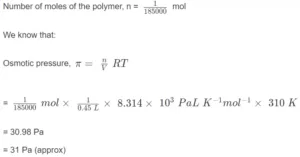Ncert Chemistry Class 12 Questions Solutions Chapter 2

NCERT
Exercise Questions with Solutions
Chapter – Solutions

Que 1. Define the term solution. How many types of solutions are formed? Write briefly about each type with an example.
Ans 1. A solution is a homogeneous mixture of two or more chemically non-reacting substances. Types of solutions: There are nine types of solutions.
Types of Solution Examples
Gaseous solutions
(a) Gas in gas Air, a mixture of 02 and N2, etc.
(b) Liquid in gas Water vapour
(c) Solid in gas Camphor vapours in N2 gas, smoke etc.
Liquid solutions
(a) Gas in liquid C02 dissolved in water (aerated water), and 02 dissolved in water, etc.
(b) Liquid in liquid Ethanol dissolved in water, etc.
(c) Solid in liquid Sugar dissolved in water, saline water, etc.
Solid solutions
(a) Gas in solid Solution of hydrogen in palladium
(b) Liquid in solid Amalgams, e.g., Na-Hg
(c) Solid in solid Gold ornaments (Cu/Ag with Au)

Que 2. Give an example of a solid solution in which the solute is a gas.
Ans 2. Solution of hydrogen in palladium and dissolved gases in minerals.

Que 3. Define the following terms:
(a) Mole fraction
(b) Molality
(c) Molarity
(d) Mass percentage.
Ans 3. (a). Mole fraction: The mole fraction of a constituent (solute as well as solvent) is the ratio of the number of moles of one component to the total number of moles of all the components present in the solution. If nA and nB are the numbers of moles of solvent and solute respectively then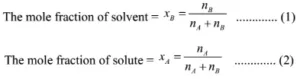(b). Molality: The molality of a solution is defined as the number of moles of the solute dissolved in one kilogram of the solvent. It is denoted by m. It is expressed as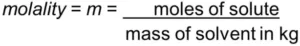(c). Molarity: The molarity of a solution is defined as the number of moles of the solute dissolved in one litre or one dm3 of the solution. It is denoted by M and is expressed as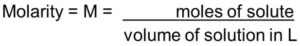(d). Mass %: It may be defined as the mass of solute in grams per 100 g of solution.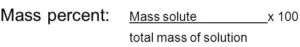Que 4. Concentrated nitric acid used in the laboratory is 68% nitric acid by mass in an aqueous solution. What should be the molarity of such a sample of the acid if the density of the solution is 1.504 g mL-1?
Ans 4. Molar mass of HNO3 = 1 + 14 + 3 × 16 = 63 g/mol
It is given that the nitric acid used in the laboratory is 68% by mass in an aqueous solution.
100 g of solution contains 68 g of nitric acid or 68/63 ​ = 1.079 moles of nitric acid.
The density of the solution is 1.504 g/mL.
100 g of solution corresponds to 100/1.504 ​ = 66.5 mL or 0.0665 L
The molarity of the solution is the number of moles of nitric acid present in 1 L of solution.
It is 1.079/0.0665 ​ = 16.22 M
Hence, the molarity should be 16.22 g/mL.

Que 5. A solution of glucose in water is labelled as 10% w/w. What would be the molality and mole fraction of each component in the solution? If the density of the solution is 1.2 g mL-1, then what shall be the molarity of the solution?
Ans 5. 10% w/w solution of glucose in water means that 10 g of glucose is present in 100 g of the solution i.e., 10 g of glucose is present in (100 – 10) g = 90 g of water.
Molar mass of glucose (C6H12O6) = 6 × 12 + 12 × 1 + 6 × 16 = 180 g mol – 1
Then, the number of moles of glucose = 10 / 180 mol
= 0.056 mol
∴ Molality of solution = 0.056 mol / 0.09kg  = 0.62 m
Number of moles of water = 90g / 18g mol-1 = 5 mol
Mole fraction of glucose  (xg) = 0.056 / ( 0.056+5) = 0.011
And, mole fraction of water x= 1 – xg
= 1 – 0.011 = 0.989
If the density of the solution is 1.2 g mL – 1, then the volume of the 100 g solution can be given as:
= 100g / 1.2g mL-1
= 83.33 mL
=83.33 x 10-3 L
∴ Molarity of the solution = 0.056 mol / 83.33 x 10-3 L
= 0.67 M

Ncert Chemistry Class 12 Questions Solutions Chapter 2

Que 6. How many mL of 0.1 M HCl are required to react completely with a 1 g mixture of Na2C0and NaHCOcontaining equimolar amounts of both?
Ans 6. Calculation of no. of moles of components in the mixture
Na2CO3 and NaHCO3 are both equimolar. It is the meaning of substances to have the same molarity
Na2CO3 in the mixture with a total amount will be x gram
NaHCO3 in the mixture with a total amount will be (1-x) gram
Now the molar mass of Na2CO3 = 106g/mol
So the number of moles is Na2CO3 = x/106mol
molar mass of NaHCO3 = 84 g/mol
Therefore, number of moles of NaHCO3 = 1 – x/84 mol
As per the given questions
x/106 = 1 – x/54
84 x = 106 – 106 x
190x = 106
x = 0.5579
Number of moles of Na2CO3 = 0.5579/106 mol
= 0.0053mol
And the number of moles of NaHCO3 = 1 – 0.5579/84
= 0.0053 mol
HCl reacts with Na2CO3 and Na2CO3 according to the following equation
2HCl + Na2CO3 ————> 2NaCl + H2O + CO2
2mol      1mol
and
HCl + NaHCO3 ———–> NaCl  + H2O + CO2
1mol     1 mol
From the above reactions,
1 mol of Na2CO3 will react with 2 mol of HCl
Therefore, 0.00526 mol of Na2CO3 will react with 2 × 0.00526 mol of HCl
similarly 0.00526 mol of NaHCO3 will react with 0.00526 mol of HCl
Total mol of HCl required to react with a mixture of NaHCO3 & Na2CO3 = 2 × 0.00526 + 0.00526= 0.01578 mol.
Que 7. A solution is obtained by mixing 300 g of 25% and 400 g of 40% solution by mass. Calculate the percentage of the resulting solution.
Ans 7. 300 g of 25% solution contains solute = 75 g
400 g of 40% solution contains solute = 160 g
Total solute = 160 + 75 = 235 g
Total solution = 300 + 400 = 700 g
% of solute in the final solution =   × 100 = 33.5%
% of water in the final solution = 100 – 33.5 = 66.5 %

Que 8. An antifreeze solution is prepared from 222.6 g of ethylene glycol, C2H4(OH)2 and 200 g of water. Calculate the molality of the solution. If the density of the solution is 1.072 g mL-1, then what shall be the molarity of the solution?
Ans 8. Calculation of Molality:
Mass of ethylene glycol = 222.6
Molar mass of ethylene glycol = 2 × 12 + 6 × 1 + 2 × 16 = 62
Therefore moles of ethylene glycol = 222.6g / 62 gmol-1 = 3.59 mol
Mass of water = 200g
Therefore Molality of the Solution = (moles of ethylene glycol / mass of water) × 1000
= (3.59 / 200) × 1000
= 17.95 m
Calculation of Molarity:
Moles of ethylene glycol = 3.59 mol
Total Mass of solution = 200 + 222.6 = 422.6g
Volume of solution = mass / density volume
= 422.6 / 1.072
= 394.22 ml
Molarity of the Solution = (moles of ethylene glycol / volume of solution) x 1000
= (3.59 / 394.22) x 1000
= 9.11 M

Que 9. A sample of drinking water was found to be severely contaminated with chloroform, CHCl3, supposed to be a carcinogen. The level of contamination was 15 ppm (by mass)
(a) Express this in per cent by mass.
(b) Determine the molality of chloroform in the water sample.
Ans 9. (a) 1 ppm is equivalent to 1 part out of 1 million (106) parts.
∴ The mass percent of 15 ppm chloroform in water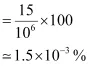(b) Molality (M) = (no of moles of solute/mass of solvent in g) *1000
Therefore mass of Chloroform= 12 + 1+3 (35.5) = 119.5 g/mol
100 g of the sample contains 1.5 × 10–3 g of CHCl3.
⇒ 1000 g of the sample contains CHCl3. = 1.5 × 10–2 g
m = (1.5 × 10-3/119.5) * 1000 = 1.25 × 10-4 m

Que 10. What role does the molecular interaction play in a solution of alcohol and water?
Ans 10. In the case of alcohol as well as water, the molecules are interlinked by intermolecular hydrogen bonding. However, the hydrogen bonding is also present in the molecules of alcohol and water in the solution but it is comparatively less than both alcohol and water. As a result, the magnitude of attractive forces tends to decrease and the solution shows a positive deviation from Raoult’s Law. This will lead to an increase in the vapour pressure of the solution and also a decrease in its boiling point.Ncert Chemistry Class 12 Questions Solutions Chapter 2

Que 11. Why do gases always tend to be less soluble in liquids as the temperature is raised?
Ans 11.
When gases are dissolved in water, it is accompanied by a release of heat energy, i.e., the process is exothermic. When the temperature is increased, according to Lechatlier’s Principle, the equilibrium shifts in the backward direction, and thus gases becomes less soluble in liquids.

Que 12. State Henry’s law and mention some of its important applications.
Ans 12. Henry’s law: The solubility of a gas in a liquid at a particular temperature is directly proportional to the pressure of the gas in equilibrium with the liquid at that temperature.
OR
The partial pressure of a gas in the vapour phase is proportional to the mole fraction of the gas (x) in the solution. p = KHX
where KH is Henry’s law constant.
Applications of Henry’s law :
(i) In order to increase the solubility of CO2 gas in soft drinks and soda water, the bottles are normally sealed under high pressure. An increase in pressure increases the solubility of a gas in a solvent according to Henry’s Law. If the bottle is opened by removing the stopper or seal, the pressure on the surface of the gas will suddenly decrease. This will cause a decrease in the solubility of the gas in the liquid i.e. water. As a result, it will rush out of the bottle producing a hissing noise or with a fizz.
(ii) As pointed above, oxygen to be used by deep-sea divers is generally diluted with helium in order to reduce or minimise the painful effects during decompression.
(iii) As the partial pressure of oxygen in the air is high, in the lungs it combines with hemoglobin to form oxyhemoglobin. In tissues, the partial pressure of oxygen is comparatively low. Therefore, oxyhaemoglobin releases oxygen in order to carry out cellular activities.

Que 13. The partial pressure of ethane over a saturated solution containing 6.56 × 10-3 g of ethane is 1 bar. If the solution contains 5.00 × 10-2 g of ethane, then what shall be the partial pressure of the gas?
Ans 13. According to Henry’s law, the solubility of a gas in a liquid is directly proportional to the pressure of X=KH​×P
X is the mole fraction of gas, KH ​is Henry’s law constant and P is the partial pressure of the gas.
Also, mole fraction of ethane will be directly proportional to its mass.
WP′=W′P
W is the mass of ethane in the first solution and P is the partial pressure of ethane in the first solution.
W′ is the mass of ethane in the second solution and P′ is the partial pressure of ethane in the second solution.
6.56×10−3×P′=5.00×10−2×1P′=7.6
Hence, the partial pressure of the gas will be 7.6 bar.

Que 14. What is meant by positive and negative deviations from Raoult’s law and how is the sign of ∆mix H related to positive and negative deviations from Raoult’s law?
Ans 14. Solutions having vapour pressures more than that expected from Raoult’s law are said to exhibit positive deviation. In these solutions, solvent-solute interactions are weaker and ∆solH is positive because stronger A – A or B – B interactions are replaced by weaker A – B interactions. Breaking of the stronger interactions requires more energy & less energy is released on the formation of weaker interactions. So overall ∆solH is positive. Similarly, ∆solV is positive i.e. the volume of solution is somewhat more than the sum of volumes of solvent and solute. So there is an expansion in volume on solution formation.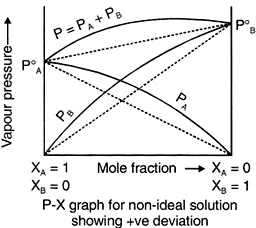Similarly, in the case of solutions exhibiting negative deviations, A – B interactions are stronger than A-A&B-B. So weaker interactions are replaced by stronger interactions so, there is the release of energy i.e. ∆sol H is negative.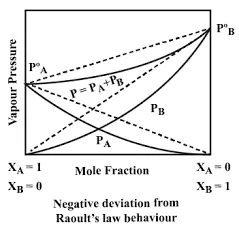Que 15. An aqueous solution of 2 per cent non-volatile solute exerts a pressure of 1·004 bar at the boiling point of the solvent. What is the molecular mass of the solute?
Ans 15. Vapour pressure of the solution at normal boiling point (p1) = 1.004 bar
Vapour pressure of pure water at normal boiling point (p10) = 1.013 bar
Mass of solute, (w2) = 2 g
Mass of solvent (water), (w1) = 100 – 2 = 98 g
Molar mass of solvent (water), (M1) = 18 g mol – 1
According to Raoult’s law,
(p10 – p1p10    = (w× M1/ (M× w1 )
(1.013 – 1.004)/1.013 = (2 × 18) / (M2 × 98)
0.009 / 1.013 = (2 × 18) / (M2 × 98)
M2 = (2 × 18 × 1.013) / (0.009 × 98)
M2 = 41.35 g mol – 1
Hence, the molar mass of the solute is 41.35 g mol – 1.

Ncert Chemistry Class 12 Questions Solutions Chapter 2

Que 16. Heptane and octane form an ideal solution. At 373 K, the vapour pressure of the two liquid components is 105.2 kPa and 46.8 kPa respectively. What will be the vapour pressure of a mixture of 26.0 g of heptane and 35.0 g of octane?
Ans 16. Vapour pressure of heptane p10 = 105.2 kPa
Vapour pressure of octane p20= 46.8 kPa
As we know that, Molar mass of heptane (C7H16) = 7 × 12 + 16 × 1 = 100 g mol – 1
∴ Number of moles of heptane = 26/100 mol = 0.26 mol
Molar mass of octane (C8H18) = 8 × 12 + 18 × 1 = 114 g mol – 1
∴ Number of moles of octane = 35/114 mol = 0.31 mol
Mole fraction of heptane, x1 = 0.26 / 0.26 +0.31 = 0.456
And, mole fraction of octane, x2 = 1 – 0.456 = 0.544
Now, partial pressure of heptane, p= xp20
= 0.456 × 105.2 = 47.97 kPa
Partial pressure of octane,p= xp20
= 0.544 × 46.8 = 25.46 kPa
Hence, vapour pressure of solution, ptotal  = p1 + p2
= 47.97 + 25.46 = 73.43 kPa

Que 17. The vapour pressure of water is 12.3 kPa at 300 K. Calculate the vapour pressure of 1 molal solution of a solute in it.
Ans 17. 1 molal solution means 1 mol of the solute is present in 1000 g of the solvent (water).
The molar mass of water = 18 g mol – 1
∴ Number of moles present in 1000 g of water = 1000/18
= 55.56 mol
Therefore, mole fraction of the solute in the solution is
x2 =  1 / (1+55.56) = 0.0177.
It is given that,
Vapour pressure of water, p1= 12.3 kPa
Applying the relation, (P1–  P1P1= X2
⇒ (12.3 – p1) / 12.3 =0.0177
⇒ 12.3 – P1 = 0.2177
⇒ p1 = 12.0823
= 12.08 kPa (approximately)
Hence, the vapour pressure of the solution is 12.08 kPa.

Ncert Chemistry Class 12 Questions Solutions Chapter 2

Que 18. Calculate the mass of a non-volatile solute (molar mass 40 g mol-1) which should be dissolved in 114 g octane to reduce its vapour pressure to 80%.
Ans 18. Let the vapour pressure of pure octane be p10.
Then, the vapour pressure of the octane after dissolving the non-volatile solute is 80/100 p10 = 0.8 p10.
Molar mass of solute, M2 = 40 g mol – 1
Mass of octane, w1 = 114 g
Molar mass of octane, (C8H18), M1 = 8 × 12 + 18 × 1 = 114 g mol – 1
Applying the relation,
(p10 – p1)/p10    =  (w× M)/(M2  × w1 )
⇒ (p10 – 0.8 p10) / p10  = (w× 114 )/(40  × 114 )
⇒ 0.2 p10 / p10   = w/ 40
⇒ 0.2 = w/ 40
⇒ w2 = 8 g
Hence, the required mass of the solute is 8 g.

Que 19. A solution containing 30g of non-volatile solute exactly in 90 g of water has a vapour pressure of 2.8 kPa at 298 K. Further, 18g of water is then added to the solution and the new of vapour pressure becomes 2.9 kPa at 298 K. Calculate (i) molar mass of the solute.
(ii) vapour pressure of water at 298 K.
Ans 19. Let, the molar mass of the solute be M g mol – 1
Now, the no. of moles of solvent (water), n= 90g 18g mol-1
And, the no. of moles of solute, n= 30g / M mol-1 = 30 / M mol
p= 2.8 kPa
Applying the relation:
(p10 – p1p10    =  n2  / (n1  + n2)
⇒ (p10 – 2.8) p10    = (30/M)  / {5  + (30/M)}
⇒ 1 – (2.8/p10)  = (30/M) / {(5M+30)/M}
⇒ 1 – (2.8/p10) = 30 / (5M + 30)
⇒ 2.8/p10  = 1 –  30 / (5M + 30)
⇒ 2.8/p10   =  (5M + 30 – 30) / (5M + 30)
⇒ 2.8/p10   = 5M / (5M+30)
⇒ p10 / 2.8  =  (5M+30) / 5M  —————-(1)
After the addition of 18 g of water:
n1 = (90+18g) / 18  = 6 mol
and the new vapour pressure is p1 = 2.9 kPa   (Given)
Again, applying the relation:
(p10 – p1p10    = n/ (n1  + n2)
⇒ (p10 – 2.9) p10   = (30/M)  / {6  + (30/M)}
⇒ 1 – (2.9/p10)  = (30/M) / {(6M+30)/M}
⇒ 1 – (2.9/p10) = 30 / (6M + 30)
⇒ 2.9/p10  = 1 –  30 / (6M + 30)
⇒ 2.9/p10  =  (6M + 30 – 30) / (6M + 30)
⇒ 2.9/p10  = 6M / (6M+30)
⇒ p10 / 2.9 =  (6M+30) / 6M  —————-(2)
Dividing equation (1) by (2),we get:
2.9 / 2.8 =  {(5M+30) / 5M} / {(6M+30) / 6M}
⇒ 2.9 × (6M+30 / 6)  =  (5M+30 / 5) ×  2.8
⇒ 2.9 ×  (6M +30) ×  5 = (5M+30) ×  2.8 ×  6
⇒ 87M + 435  =  84M + 504
⇒ 3M = 69
⇒ M = 23u
Therefore, the molar mass of the solute is 23 g mol -1.

(ii) Putting the value of ‘M’ in equation (i), we get:
⇒ p10 / 2.8  =  (5M+30) / 5M
⇒ p10 / 2.8  =  (5×23+30) / 5×23
⇒  p10  =  (145 × 2.8) / 115
⇒  p10  =  3.53
Hence, the vapour pressure of water at 298 K is 3.53 kPa.

Que 20. A 5% solution (by mass) of cane sugar in water has a freezing point of 271 K. Calculate the freezing point of 5% glucose in water if the freezing point of pure water is 273.15 K.
Ans 20. In case of cane sugar:
ΔT= (273.15 – 271) K = 2.15 K
Molar mass of sugar (C12H22O11) = 12 × 12 + 22 × 1 + 11 × 16 = 342 g mol – 1
5% solution (by mass) of cane sugar in water means 5 g of cane sugar is present in (100 – 5)g = 95 g of water.
Now, number of moles of cane sugar =5/342 mol
= 0.0146 mol
Therefore, molality of the solution, m =0.0146mol / 0.095kg
= 0.1537 kg mol – 1
Now applying the relation,
ΔTf = Kf × m
= Kf =  ΔTf  / m
=  2.15K / 0.1537 kg mol-1
= 13.99 K kg mol-1
Molar of glucose (C6H12O6) = 6 × 12 + 12 × 1 + 6 × 16 = 180 g mol – 1
5% glucose in water means 5 g of glucose is present in (100 – 5) g = 95 g of water.
∴ Number of moles of glucose = 5/180 mol
= 0.0278 mol
Therefore, molality of the solution,m =0.0278 mol / 0.095 kg
= 0.2926 mol kg – 1
Applying the relation,
ΔTf = Kf × m
= 13.99 K kg mol – 1 × 0.2926 mol kg – 1
= 4.09 K (approximately)
Hence, the freezing point of the 5% glucose solution is (273.15 – 4.09) K= 269.06 K.

Que 21. Two elements A and B form compounds having formula AB2 and AB4. When dissolved in 20g of benzene (C6H6), 1 g of AB2 lowers the freezing point by 2.3 K whereas 1.0 g of AB4 lowers it by 1.3 K. The molar depression constant for benzene is 5.1 K kg mol-1. Calculate atomic masses of A and B.
Ans 21. As We know that:
MB = (Kf × wB × 1000) / (wA × ΔTf)
Now ΔTf = 2.3 , wB = 1.0 , wA = 20, Kf = 5.1 (given)
Putting the values in the equation
MB = (5.1 × 1 × 1000) / (20 × 2.3) = 110.87 g/mol
Therefore MAB2 = 110.9
For AB4 compound
ΔTf = 1.3, wb = 1, wa = 20
MB = (5.1 × 1 × 1000) / (20 × 1.3) = 196 g/mol
Therefore MAB = 196
Let x be the atomic mass of A & y be the atomic mass of B,
Then MAB2 = x + 2y = 110.9 ———————————-(1)
And MAB = x + 4y = 196        ———————————-(2)
Subtracting 2 from 1, we get
2y = 196 – 110.9
y = 85.1 / 2
y = 42.6
Putting the value of y in 1 we get
x = 110.9 – 2 × 42.6
x = 25.59
Therefore the atomic mass of A = 25.59 u Atomic mass of B = 42.6 u.

Ncert Chemistry Class 12 Questions Solutions Chapter 2

Que 22. At 300 K, 36 g glucose present per litre in its solution has an osmotic pressure of 4·98 bar. If the osmotic pressure of the solution is 1·52 bar at the same temperature, what would be its concentration?
Ans 22. π1= 4.98
π2 = 1.52
C1 = 36/180
C2 = ? (we have to find)
Now according to van’t hoff equation
Π = CRT
Putting the values in above equation, we get
4.98 = 36/180RT ————————1
1.52 = c2RT       ————————2
Now dividing equation 2 by 1, we get
(c2 × 180) / 36 = 1.52 / 4.98
or
c2 = 0.061
Therefore the concentration of the 2nd solution is 0.061 M.

Que 23. Suggest the most important type of intermolecular attractive interaction in the following pairs:
(i) n-hexane and n-octane
(ii) I2 and CCl4.
(iii) NaClO4 and water
(iv) methanol and acetone
(v) acetonitrile (CH3CN) and acetone (C3H6O).
Ans 23. (i) Both n-hexane and n-octane are non-polar. Thus, the intermolecular interactions will be London dispersion forces.
(ii) Both I2 and CCl4 are non-polar. Thus, the intermolecular interactions will be London dispersion forces.
(iii) NaClO4 is an ionic compound and gives Na+ and ClO4 ions in the Solution. Water is a polar molecule. Thus, the intermolecular interactions will be ion-dipole interactions.
(iv) Both methanol and acetone are polar molecules. Thus, intermolecular interactions will be dipole-dipole interactions.
(v) Both CH3CN and C3H6O are polar molecules. Thus, intermolecular interactions will be dipole-dipole interactions.

Ncert Chemistry Class 12 Questions Solutions Chapter 2

Que 24. Based on solute-solvent interactions, arrange the following in order of increasing solubility in n-octane and explain. Cyclohexane, KCl, CH3OH, CH3CN.
Ans 24. n-octane (C8H18) is a non-polar liquid and solubility is governed by the principle that like dissolve like. Keeping this in view, the increasing order of solubility of different solutes is:
KCl < CH3OH < CH3C=N < C6H12 (cyclohexane).

Que 25. Amongst the following compounds, identify which are insoluble, partially soluble and highly soluble in water?
(i) phenol
(ii) toluene
(iii) formic acid
(iv) ethylene glycol
(v) chloroform
(vi) pentanol
Ans 25. (i) Phenol (having polar – OH group) – Partially soluble.
(ii) Toluene (non-polar) – Insoluble.
(iii) Formic acid (form hydrogen bonds with water molecules) – Highly soluble.
(iv) Ethylene glycol (form hydrogen bonds with water molecules) Highly soluble.
(v) Chloroform (non-polar)- Insoluble.
(vi) Pentanol (having polar -OH) – Partially soluble.

Que 26. If the density of lake water is 1·25 g mL-1, and it contains 92 g of Na+ ions per kg of water, calculate the molality of Na+ ions in the lake.
Ans 26. Molality = moles of solute / mass of solvent in kg
Now mass of Na+ ions = 92 g (given)
Moles of Na+ ions = 92 / 23 = 4
And mass of water = 1kg
Therefore molality = 4/1 = 4M

Que 27. If the solubility product of CuS is 6 x 10-16, calculate the maximum molarity of CuS in aqueous solution.
Ans 27. Solubility product of CuS, Ksp of CuS = 6 x 10-16
If s is the solubility,
then CuS = cu2+ + S2-
Therefore KSP = { cu2+}{ S2-}
Or
KSP = s × s
Or
s = √ KSP = √6 × 10-16
= 2.45 × 10-8 M

Que 28. Calculate the mass percentage of aspirin (C9H8O4 in acetonitrile (CH3CN) when 6.5g of CHO is dissolved in 450 g of CH3CN.
Ans 28.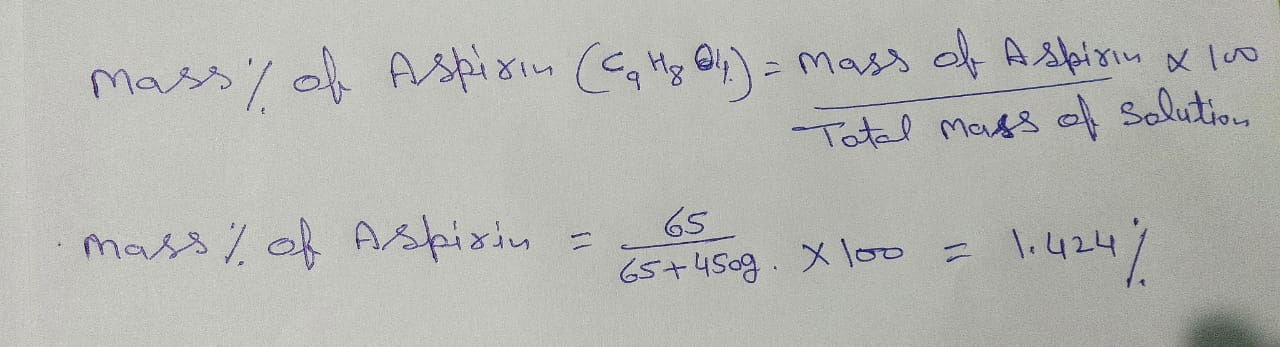Que 29. Nalorphene (C19H21NO3), similar to morphine, is used to combat withdrawal symptoms in narcotic users. Dose of nalorphene generally given is 1.5 mg. Calculate the mass of 1.5 x 10-3 m aqueous solution required for the above dose.
Ans 29.
We are given with molality of the solution, so we need to find the moles of Nalorphene.
Molar mass of nalorphene =  19(12) + 21(1)  + 1(14) + 3(16)  =  311u.
So moles of nalorphene: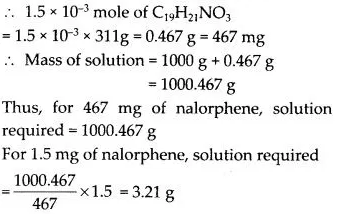Que 30. Calculate the amount of benzoic acid (C5H5COOH) required for preparing 250 mL of 0· 15 M solution in methanol.
Ans 30. 0.15 M solution means that 0.15 moles of C6H5COOH is present in 1L = 1000 mL of the solution
Molar mass of C6H6COOH = 72 + 5 + 12 + 32 + 1 = 122 g mol-1
Thus, 1000 mL of solution contains benzoic acid = 18.3 g
250 mL of solution will contain benzoic acid = (18.3/1000)250 = 4.575 g.

Que 31. The depression in the freezing point of water observed for the same amount of acetic acid, trichloroacetic acid and trifluoroacetic acid increases in the order given above. Explain briefly.
Ans 31. Fluorine, being the most electronegative, has the strongest electron-withdrawing inductive effect (-I effect). Consequently, trifluoroacetic acid is the strongest acid while acetic acid is the weakest acid. Hence, trifluoroacetic acid ionizes most while acetic acid ionizes least. The more the number of ions produced, the more is the depression in the freezing point. Thus, the depression in freezing point is maximum for the trifluoroacetic acid and minimum for acetic acid. The depression in freezing points are in the order: Acetic acid < trichloroacetic acid < trifluoracetic acid.

Que 32. Calculate the depression in the freezing point of water when 10g of CH3CH2CHClCOOH is added to 250g of water. Ka = 1.4 × 1o-3 Kg = 1.86 K kg mol-1.
Ans 32. Molar mass of CH3CH2CHClCOOH
15 + 14 + 13 + 35.5 + 12 + 16 + 16 + 1
= 122.5 g/mol
∴ Moles of CH3CH2CHClCOOH = 10g / 122.5 g/mol
= 0.0816 mol
Therefore molality of the solution
= (0.0816 × 1000) / 250
= 0.3265 mol kg-1
Now if a is the degree of dissociation of CH3CH2CHClCOOH,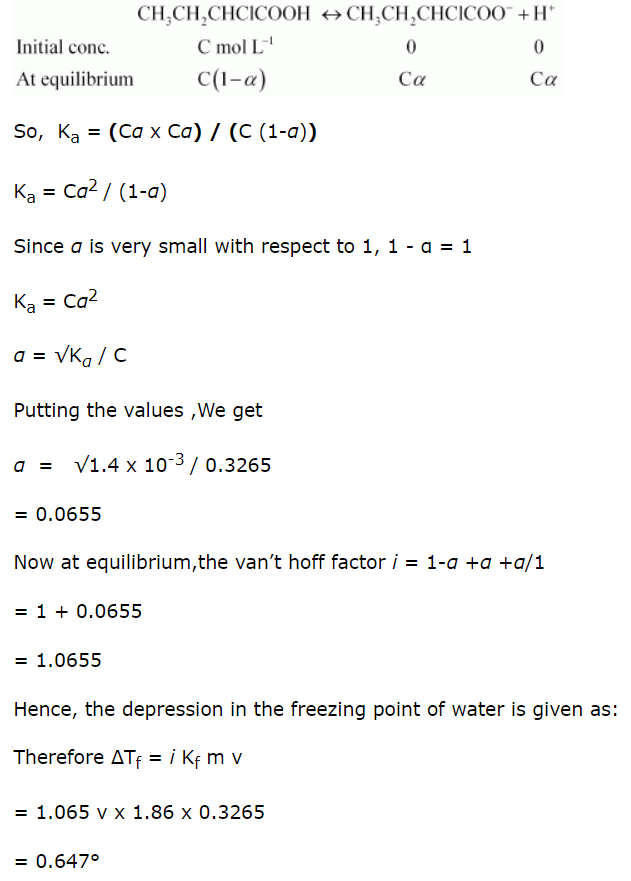Que 33. 19.5g of CH2FCOOH is dissolved in 500g of water. The depression in the freezing point of water observed is 1.0°C. Calculate the van’s Hoff factor and dissociation constant of fluoroacetic acid.
Ans 33. Molecular mass of CH2FCOOH
14 + 19 + 12 + 16 + 16 + 1 = 78 g/mol
Now, Moles of CH2FCOOH = 19.5 / 78 = 0.25
Taking the volume of the solution as 500 mL, we have the concentration:
C = (0.25 / 500) × 1000
Therefore Molality =  0.50m
So now putting the value in the formula:
ΔTf = Kf × m
=1.86 × 0.50 = 0.93K
van’t Hoff factor = observed freezing point depression / calculated freezing point depression
= 1 / 0.93 = 1.0753
Let α be the degree of dissociation of CH2FCOOH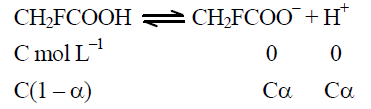Now total number of moles = C(1-α) + C α +Cα = C(1 + α)
Or
i = α(1+α) / α = 1 +α  = 1.0753
Therefore α = 1.0753- 1 = 0.0753
Now the Value of Ka is given as:
Ka  = [CH2FCOO][H+]  /  CH2FCOOH
(Cα × Cα) / (C (1-α))
= Cα/ (1-α)
Ka = 0.5 × (0.0753)2  /  (1-0.0753)
= 0.5 × 0.00567 0.09247
= 0.00307 (approx.)
= 3 × 10-3

Que 34. Vapour pressure of water at 293 K is 17·535 mm Hg. Calculate the vapour pressure of water at 293 K when 25 g of glucose is dissolved in 450 g of water.
Ans 34. Vapour pressure of water, p1° = 17.535 mm of Hg
Mass of glucose, w2 = 25 g
Mass of water, w1 = 450 g
We know that,
The molar mass of glucose (C6H12O6),
M= 6 × 12 + 12 × 1 + 6 × 16 = 180 g mol – 1
The molar mass of water, M1 = 18 g mol – 1
Then, number of moles of glucose, n1 = 25/180 = 0.139 mol
And, number of moles of water, n2 =450/18 = 25 mol
Now, we know that,
(p1° – p°) / p1° =  n1 / n2 + n1
⇒ 17.535 – p°  / 17.535 =   0.139 / (0.139+25)
⇒ 17.535 – p1 = 0.097
⇒ p1 = 17.44 mm of Hg
Hence, the vapour pressure of water is 17.44 mm of Hg.

Que 35. Henry’s law constant for the molality of methane in benzene at 298 K is 4.27 × 105 mm Hg. Calculate the solubility of methane in benzene at 298 K under 760 mm Hg.
Ans 35. As we know that
p = k × c
We have given p = 760 mm, k = 4.27 × 105
Putting the given values in equation
760 = 4.27 × 10× c
Or
c = 760 / 4.27 × 105
c = 178 × 10-5.

NCERT Class 12 Chemistry Chapter 2 Exercise Solutions

Que 36. 100g of liquid A (molar mass 140 g mol-1) was dissolved in 1000g of liquid B (molar mass 180g mol-1). The vapour pressure of pure liquid B was found to be 500 torr. Calculate the vapour pressure of pure liquid A and its vapour pressure in the solution if the total vapour pressure of the solution is 475 torr.
Ans 36. Number of Moles of Liquid A, nA = 100 / 140 = 0.714
Number of Moles of Liquid B, n= 1000 / 180 = 5.556
Then Mole fraction of A = nA / nA + nB = 0.714 / 0.714 + 5.556 = 0.114
Now Mole of fraction of B = 1 – 0.114 = 0.886
Now ptotal  = pA + pB
Or ptotal = p°AXA + p°BxB
OR
475 = p°A × 0.114 + 500 × 0.886
OR
A = 280.7 torr
Therefore vapour pressure of pure A = 280.7 torr
Vapour pressure of A in solution = 280.7 × 0.114 = 32 torr
Now
pA  = p°AXA
Or
A  = pA   /  XA
⇒ 32 / 0.114
= 280.7 torr
Hence, the vapour pressure of pure liquid A is 280.7 torr.

Que 37. Vapour pressure of pure acetone and chloroform at 328 K are 741.8 mm Hg and 632.8 mm Hg respectively. Assuming that they form ideal solution over the entire range of composition, plot Ptotal, Pchloroform, and Pacetone as a function of Xacetone. The experimental data observed for different compositions of the mixture is

 100 ×xacetone 0 11.8 23.4 36 50.8 58.2 64.5 72.1 pacetone /mm Hg 0 54.9 110.1 202.4 322.7 405.9 454.1 521.1 pchloroform/mm Hg 632.8 548.1 469.4 359.7 257.7 193.6 161.2 120.7

Ans 37.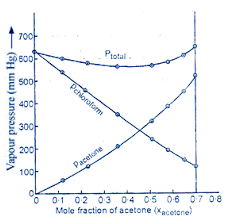Que 38. Benzene and toluene form ideal solutions over the entire range of composition. The vapour pressure of pure benzene and toluene at 300 K are 50.71 mm Hg and 32.06 mm Hg respectively. Calculate the mole fraction of benzene in vapour phase if 80g of benzene is mixed with 100g of toluene.
Ans 38. Molar mass of benzene (C6H6) = 6 × 12 + 6 × 1 = 78 g/mol
Molar mass of toluene = 7 x 12 + 8 × 1 = 92 g/mol
Now no of moles in 80g of benzene = 80 / 78 = 1.026 mol
No of moles in 100g of toluene = 100 / 92 = 1.087 mol
∴Mole fraction of benzene xb = 1.026 / 1.026 + 1.087 = 0.486
And Mole fraction of toluene, xt = 1 – 0.486 = 0.514
We have given that
Vapor pressure of pure benzene pb° = 50.71 mm Hg
And, vapour pressure of pure toluene, pt° = 32.06 mm Hg
Therefore partial Vapor pressure of benzene, pb = pb° × xb
= 50.71 × 0.486
= 24.65 mm Hg
And partial Vapor pressure of toluene, pt = pt × xt
Pt = p°t × xt = 32.06 × 0.514
= 16.48
Total vapour pressure = 24.65 + 16.48 = 41.13 mm Hg
Mole fraction of benzene in vapour phase = 24.65 / 41.13 = 0.60.

NCERT Class 12 Chemistry Chapter 2 Exercise Solutions

Que 39. The air is a mixture of a number of gases. The major components are oxygen and nitrogen with an approximate proportion of 20% is to 79% by volume at 298 K. The water is in equilibrium with air at a pressure of 10 atm. At 298 K if Henry’s law constants for oxygen and nitrogen are 3.30 × 107 mm and 6.51 × 107 mm respectively, calculate the composition of these gases in water.
Ans 39. Percentage of oxygen (O2) in air = 20%
Percentage of nitrogen (N2) in air = 79%
Also, it is given that water is in equilibrium with air at a total pressure of 10 atm,
that is, (10 × 760) mm Hg = 7600 mm Hg
Therefore, Partial pressure of oxygen, pO2 = (20/100)7600 =1520 mm Hg
Partial pressure of nitrogen, pN2 = (79/100)7600 = 6004 mmHg
Now, according to Henry’s law:
p = KH.x
For oxygen:
pO2  = KH. xO2
xO2 po2 / KH
= 1520 / 3.30 × 107
= 4.61 10-5
For nitrogen
pN2 = KH.xN2
xN2 pN2 / KH
= 6004 / 6.51 × 107
= 9.22 × 10-5
Hence, the mole fractions of oxygen and nitrogen in water are 4.61 ×10-5 and 9.22 × 10-5 respectively.

Que 40. Determine the amount of CaCl2 (i = 2.47) dissolved in 2.5 litres of water such that its osmotic pressure is 0.75 atm at 27°C.
Ans 40. We know that
π = i n/V RT
⇒ π = i w/MV iRT
⇒ w = πMV /  iRT     …………………..(1)
Now we have given below values:
π = 0.75 atm
V = 2.5L
i = 2.47
T = (27 + 273) K = 300K
Here,
R = 0.0821 L atm k-1 mol-1
M = 1 × 40 + 2 × 35.5 = 111 g/mol
Now putting the value in equation 1
w = 0.75 × 111 × 2.5 2.47 × 0.0821 × 300 =3.42g
Hence, the required amount of CaCl2 is 3.42 g.

Ncert Chemistry Class 12 Questions Solutions Chapter 2